# GED Math : Supplementary Angles

## Example Questions

1 2 3 4 6 Next →

### Example Question #51 : Supplementary Angles

Find the measure of angle B if it is the supplement to angle A: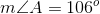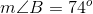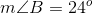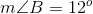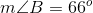Explanation:

If two angles are supplementary, that means the sum of their degrees of measure will add up to 180. In order to find the measure of angle B, subtract angle A from 180 like shown: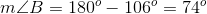This gives us a final answer of 74 degrees for angle B.

### Example Question #52 : Supplementary Angles

Find the measure of angle B if it is the supplement to angle A: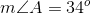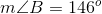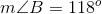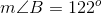Explanation:

If two angles are supplementary, that means the sum of their degrees of measure will add up to 180. In order to find the measure of angle B, subtract angle A from 180 like shown: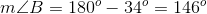This gives us a final answer of 146 degrees for angle B.

### Example Question #53 : Supplementary Angles

Find the measure of angle B if it is the supplement to angle A: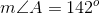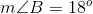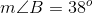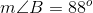Explanation:

If two angles are supplementary, that means the sum of their degrees of measure will add up to 180. In order to find the measure of angle B, subtract angle A from 180 like shown: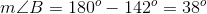This gives us a final answer of 38 degrees for angle B.

### Example Question #54 : Supplementary Angles

Find the measure of angle B if it is the supplement to angle A: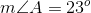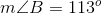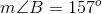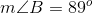Explanation:

If two angles are supplementary, that means the sum of their degrees of measure will add up to 180. In order to find the measure of angle B, subtract angle A from 180 like shown: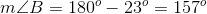This gives us a final answer of 157 degrees for angle B.

### Example Question #51 : Supplementary Angles

Find the measure of angle B if it is the supplement to angle A: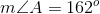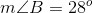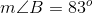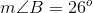Explanation:

If two angles are supplementary, that means the sum of their degrees of measure will add up to 180. In order to find the measure of angle B, subtract angle A from 180 like shown: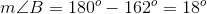This gives us a final answer of 18 degrees for angle B.

### Example Question #56 : Supplementary Angles

Find the measure of angle B if it is the supplement to angle A: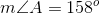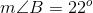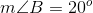Explanation:

If two angles are supplementary, that means the sum of their degrees of measure will add up to 180. In order to find the measure of angle B, subtract angle A from 180 like shown: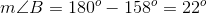This gives us a final answer of 22 degrees for angle B.

1 2 3 4 6 Next →

### All GED Math Resources